# Using a New Combination of P&O and ICM Methods for the Experimental Validation of MPPT Efficacy

Using a New Combination of P&O and ICM Methods for the Experimental Validation of MPPT Efficacy
Energy and Renewable Energy Department, Faculty of Engineering, Egyptian Chinese University, Cairo 11724, Egypt

Corresponding Author Email:
ahmedabdelbaset2016@gmail.com
Page:
797-804
|
DOI:
https://doi.org/10.18280/jesa.540601
6 September 2021
|
Accepted:
19 November 2021
|
Published:
29 December 2021
| Citation

OPEN ACCESS

Abstract:

All over the world, photovoltaic (PV) systems have now become a hopeful way to obtain transition to sustainable energy. Under different operating conditions, the voltage and maximum power generated by PV arrays can differ. The present study aims to employ a PV experimental model to propose a technique for tracking the maximum power point using a new control method for DC-DC converters. The Perturb and Observe (P&O) and Incremental Conductance Method (ICM) were combined to obtain a new combination method. The test system comprises a PV model, a DC-DC converter, a battery, an inverter, and a load. Simulation and experimental results that are totally compatible with each other demonstrate that the devised method can be used as a platform for the PV maximum power point tracking (MPPT) systems.

Keywords:

maximum power point tracking (MPPT), perturb and observe (P&O), incremental conductance method (ICM)

1. Introduction

Nowadays, solar energy is the third most widely used renewable power source after hydro and wind energy . With numerous photovoltaic power stations around the world, PV systems' most distinguishing features are a low-carbon impression and energy recovery time . With the developments achieved in 2013, power produced globally from PV systems rose to 138.9 GW [3, 4]. Later developments in 2015 further increased total energy production to 229.3 GW [3, 4]. By 2017, cumulative installed PV capacity hit approximately 400 GW. It is expected that energy produced from these systems will have increased up to 4500 GW by 2050 . Nevertheless, with the improvements in solar panel technology, there are attempts in progress to maximize energy efficiency and minimize the cost of power production .

Given the power-voltage features of PV arrays and the challenges in estimating power generation capacities and creating systems to maximize power production, such as MPPT, building an error-free and trustworthy solar array model to be used in PV systems becomes necessary. The most important issue with building error-free arrays is usually the non-linear relationship between the current and resulting voltage, especially under different operating conditions such as partial shading effects.

MPPT is a control system used by inverters, solar battery chargers, and similar devices to generate MP from PV systems. When the radiation distribution is uniform to the system, there is one MPP per radiation state due to the non-linear relationship between $I_{p v}$ and $V_{p v}$ in a PV unit . An MPPT system aims at ensuring that the system is running in the MPP, in order to produce as much power from the PV array as possible.

PV arrays work under irradiance conditions that change slowly, and MPPTs use conventional MPPT techniques, for instance, P&O and Inc. Cond. (IC). If PV systems work under partial shading effects, the P-V curve indicates several close maximum limits, which may cause the traditional MPPT to halt, leading to power loss (about 70%) .

Various criteria, such as direct search strategy, intelligent search strategy, and compensation technique are used to categorize MPPT techniques for PV systems. Various $I_{p v}$ and $V_{p v}$ systems have been introduced in recent years . The direct search strategy measures the actual I, V, and P directly from the ends of the PV array, and determines the $V_{r e f}$. A direct search strategy can be easily implemented, but sometimes, the system is located at the local maximum point (LMP). To search for the MPP, intelligent search strategies employ artificial intelligence-based methods, such as artificial neural networks (ANN) , particle swarm optimization (PSO) , genetic algorithm (GA) , fuzzy logic , or a combination of two or more of these methods . In this chapter, we investigate MPPT techniques according to their capacity to track global MPP.

## 1.png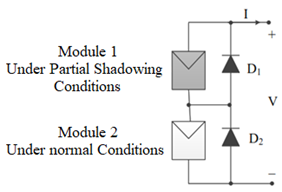Figure 1. A group of two series PV modules, $M_{1}$ under partial shadowing conditions and $M_{2}$ under normal radiation

To demonstrate the mechanism of power loss due to partial shading effects, two PV modules connected in series are checked as illustrated in Figure 1. Two maxima $\left(G_{1}\right.$ and $\left.G_{2}\right)$ in the resulting P-V curve can be seen on the output power curve $2\left(P_{2}\right)$, as illustrated in Figure 2 . When uniform radiological conditions are applied to this group, only one MPP $\left(G_{3}\right)$ is found on the output power curve $1\left(P_{1}\right)$, as illustrated in Figure 2. To ensure that the PV array generates more power, the system must operate on MPP ($G_{3}$ or $G_{1}$, depending on the radiation level).

## 2.pngFigure 2. The P-V curve for the two connected groups

Generally, partial shading makes the shaded units turn around one side and makes them act as a load instead of a generator. Thus, energy gets lost in the form of heat. This both results in one of the major reasons for energy loss in a PV unit and may cause increased temperature spots known as hotspots . The entire PV system may even receive damages from the heat caused by hotspots in the MP. To eliminate this problem, external bypass diodes are added to the PV module to help the overcurrent produced by the unshaded units move through them . Thus, hotspots are prevented from occurring. Blocking diodes are also utilized to block current flow from the battery bank to the PV array.

The present study aims to eliminate the problems of PV systems in the electrical network by evaluating the performance of a PV system. By improving all MPPT techniques to work under diverse conditions and attenuating their adverse effects particularly because of environmental fluctuations, the output power of these systems can be increased. Accordingly, the aims of the present study can be listed as follows:

To examine different MPPT methods.

To introduce a modified MPPT method.

To test the PV system using the proposed modified MPPT method.

The rest of this paper is organized as follows: Part 2 discusses photovoltaic modeling. Part 3 describes in detail the MPPT methodologies used. Part 4 shows the results of the experiment carried out to verify the modified technique, and the last section is the conclusion.

2. PAGE Setup Modeling of Solar PV and the System

## 3.pngFigure 3. Equivalent circuit for single diode (SDM) model of PV cell

The equivalent circuit of a PV cell is shown in Figure 3, in which the modest model can be represented by a current source connected parallel with SDM and the non-idealities are represented by series $\left(R_{s}\right)$ and parallel $\left(R_{p}\right)$ resistors. The mathematical equation of the yield current of a single PV model can be written as follows :

$I=I_{p h}-I_{r}\left[e^{\frac{q\left(V+I R_{s}\right)}{\eta k T}}-1\right]-\frac{V+I R_{s}}{R_{p}}$      (1)

where, $V$ denotes the yield voltage of one PV panel, $I_{p h}$ the photocurrent, $I_{r}$ the saturation current, $q$ the electrical charge $\left(1.6 \times 10^{-19} C\right), \eta$ the $p-n$ junction quality factor, $k$ the Boltzmann constant $\left(1.38 \times 10^{-23} \mathrm{~J} / \mathrm{K}\right)$, and $T$ the temperature (in kelvins ${ }^{\circ} \mathrm{K}$ ).

2.1 I-V characteristics of PV

Table 1 shows all the parameters of the PV module that will be used to implement I-V characteristics of the PV system.

Table 1. Electrical performance of the VS-100P module

 Parameters Value Voltage at $M P P, V_{m p}$ 17.8 V Current at $M P P, I_{m p}$ 5.62 A Power at $M P P, P_{m p}$ 100 W Open circuit voltage, $V_{O C}$ 21.6 V Short circuit current, $I_{S C}$ 5.97 A

Figure 4 represents the I-V characteristics of the module given in Table 1. The characteristics are observed at std.

## 4.pngFigure 4. I-V and P-V curves of one module at 25℃

2.2 System understudy

Using the MATLAB/Simulink, the entire system is simulated (as illustrated in Figure 5), and under different operating conditions (solar irradiance and temperature), the ICM-P&O-based MPPT technique will be used to identify the maximum power.

## 5.pngFigure 5. Block diagram for the studied system

3. MPPT Algorithms

MPPT is a control feature for PV systems that tracks the maximum point in order to maximize the yield of the PV unit. Environmental conditions and temperature variations affect MPPT methods since there is a relationship between temperature and irradiance levels. Therefore, irradiance levels must be taken into consideration in MPPT methods. Thus, $P_{m p p}=V_{m p p} \times I_{m p p}$. A number of algorithms and methods have been introduced to ensure that the PV system yields maximum output power based on radiation and application . While some methods achieve maximum power (MP) directly, some do not. Indirect tracking methods measure the present voltage and develop mathematical calculations to approximate the MP value; as a result, they aren't regarded actual tracking techniques. Therefore, such low-cost, simple indirect methods are often influenced by environmental factors. Direct techniques, on the other hand, are the result of extensive research because they do not require a vast memory or database. Until 2007, very few investigations had been performed on MPPT methods. However, later, many MPPT methods were created, including linearization, fuzzy control, Hybrid MPPT, artificial neural network techniques, etc. [17, 18].

3.1 Perturb and observe method

## 6.pngFigure 6. The P-V curve illustrates the perturbation direction of the voltage

## 7.png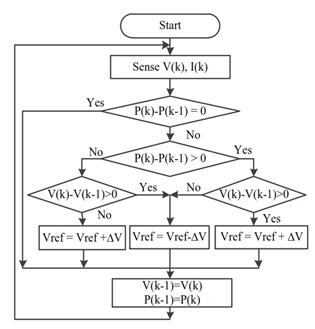Figure 7. Flowchart of $P \& O M P P T$ 

$\mathrm{P} \& \mathrm{O}$ is based on the continuity of the perturb by increasing or decreasing the $V_{p v}$ and comparing the instantaneous power $P_{k}$ and the previous one $P_{k-1}$, to determine the direction of the next stage. At the point where the perturbation causes a rise in the yield power, the current perturbation direction of the next perturbation is maintained; otherwise, the perturbation’s direction is reversed . For example, assume that the perturbation starts from point (A) in Figure 6. Following a positive voltage perturbation, increased power is observed at (B). The next voltage perturbation will thus follow a similar path as the previous one, which indicates an increase in the reference voltage. By repeating periodically, the trigger point (C) will be at MPP, but oscillations will occur. Also, in other cases, the operating point will be reached (C) . Figure 7 demonstrates the flowchart of the traditional P&O technology.

3.2 Incremental Conductance Method (ICM)

ICM is based on the principle that the throughput power derivative (P), in terms of voltage (V), at the maximum power point (MPP) is zero $(d P / d V=0)$ . Thence, from the equation $(P=I V)$, the following equation is acquired :

$\frac{d P}{d V}=V \frac{d I}{d V}+I=0($ at $M P P)$      (2)

Thus, within a single sampling period, Eq. (2) can be modified as follows:

$\frac{\Delta I}{\Delta V}=-\frac{I}{V}$      (3)

According to Eq. (2), at MPP, the PV array's instantaneous conductivity on the left side of the equation rises to the additional conductivity on the right side. For this reason, the derivative of points must be above and below zero on the left side (point C as shown in Figure 6) and on the right side of the MPP, respectively. These are explained as follows in [7, 16]:

If $\frac{d P}{d V}=0\left(\frac{d I}{d V}=-\frac{I}{V}\right)$, then MPP is reached.      (4)

If $\frac{d P}{d V}>0\left(\frac{d I}{d V}>-\frac{I}{V}\right)$, then increase $V_{\text {ref }} .$      (5)

If $\frac{d P}{d V}<0\left(\frac{d I}{d V}<-\frac{I}{V}\right)$, then decrease $V_{\text {ref }} .$      (6)

## 8.png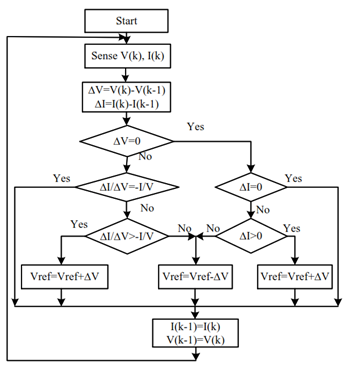Figure 8. The flowchart of the ICM MPPT 

Figure 8 demonstrates the flowchart of the IC. This methodology begins with the $V$ and $I$ qualities detected and calculates $V$ and $I$ changes compared to past advances. If the value of the $\Delta V$ is zero and the $\Delta I$ is non-zero, at this stage, the direction of the voltage changes in the next stage, which will increase when $\Delta I$ is greater than zero or decrease when $\Delta I$ is below zero. On the other hand, the direction of the voltage changes depending on Eqns. (5) and (6) to detect the required output voltage $\left(V_{\text {ref }}\right)$ from the PV system.

Comparisons of the efficiency of IC and P&O techniques [7, 8] have revealed that IC outperforms P&O.

3.3 ICM-P&O - based MPPT

One of the topics the current study deals with is the ICM-based P&O algorithm (ICM-P&O), which is a combination of two algorithms, as the name suggests. In this technique, the P&O technique determines the initial conditions of ICM and then feeds them to it. These initial conditions enable speedy MPP tracking with great accuracy and no oscillations. In this technique, the P&O operates directly while ICM remains in standby mode until the P&O decides the initial conditions.

Generally, the P&O technique is used to determine the ICM's initial duty cycle (D). The technique uses current and voltage sensors to monitor and analyze the PV system's efficiency. Since they rely on passive circuitry to test current and voltage, these two methods in combination are less difficult to obtain. The measured values should be recorded to demonstrate any increase or decrease in the duty cycle. By comparing the current input power with the previous input power, it can be decided whether there has been an increase or decrease in the (D) to keep tracking the MPP .

ICM, which is based on gradual measurements of the modification in the PV system, is another technique for tracking MPP. These data can be used to determine if the power has increased or decreased. The IC is deﬁned as $\left(d I_{P V} / d V_{P V}\right)$. This measurement can be compared to the actual conductance of the PV array to determine on which side of the MPP the present operating point is located. The duty cycle generated by the P&O algorithm is fed as an initial condition to the ICM algorithm, giving the ICM the conditions necessary for tracking MPP effectively. The entire system produces its duty ratio after a considerable amount of time, and the duty ratio feeds the DC-DC converter that affects the yield control either by an increase or decrease. The flowchart of the ICM-based P&O technique is shown in Figure 9 .

When D is adjusted after a specified period, the built-in system verifies the recently measured input power. Once the new input power (and voltage) value is bigger than the previous value, D drops to approximate to the greatest power. Besides, if the previous value is greater than the new input voltage value, and if there is more input power, then D is increased. On the other hand, if the new power value is lower than the previous value, and if the new voltage value is bigger than the previous one, D decreases to converge to MPP. Also, if the previous current power and voltage values are bigger than the new ones, D decreases .

Ultimately, by using the ICM combination and the precision of P&O technology, MPP is achieved at high speed without any oscillations or distortions.

## 9.png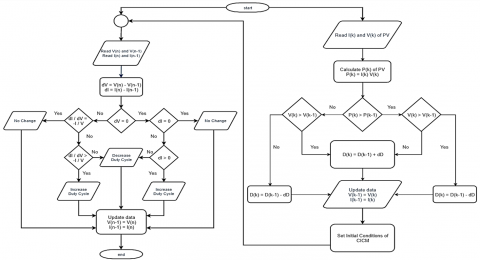Figure 9. Flowchart of the ICM-P&O-based MPPT method 

4. Simulation Results

The following figures demonstrate the output waveforms of a PV system comprising a 100W PV panel, a 12V 100Ah battery, with an MPPT with an ICM-P&O method connected to a buck converter that manages the battery charge.

Figure 10 illustrates the output voltage and output power waveforms of the PV under constant temperature and radiation varies as shown. It also presents the duty cycle waveform that generates due to the use of the suggested method that manages the DC converter.

## 10.pngFigure 10. Output power, voltage due to duty cycle via time for changing in irradiance of PV using ICM-P&O based MPPT

## 11.png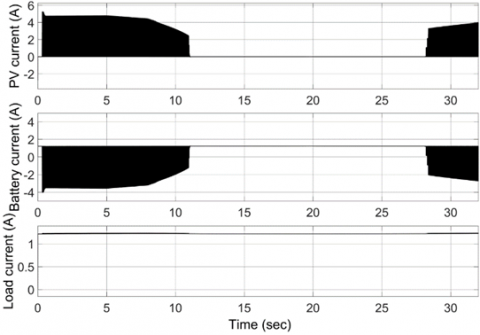Figure 11. Output waveforms of currents of PV, battery, and load

## 12.png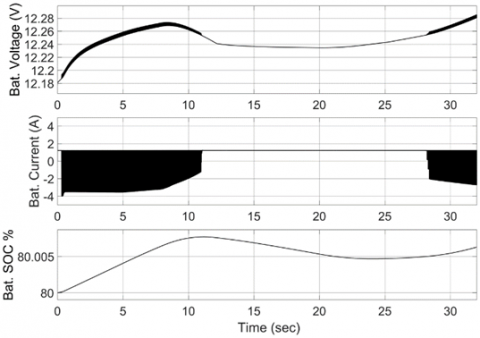Figure 12. Output waveforms of voltage and current of the battery and its SOC

Figure 11 demonstrates the yield currents from the PV and the battery used to feed the load. It obviously demonstrates that the load current is equal to the sum of the photovoltaic and battery currents, the buck converter operates in a non-continuous temperament process. It also shows that the average photovoltaic voltage is positive, while the average voltage of the battery is negative, so the PV can save the load while charging the battery.

According to Figure 12, the battery can be the source of the circuit and can provide the load when the PV is out of service. The figure also shows the output waveform of the voltage and current of the battery being used, as well as the state of discharge (SOC) percentage of the battery.

As illustrated in the figures above, the proposed technique achieves a successful tracking of the MPP of the photovoltaic system even under radiation variation, with an average efficiency of 96 percent. In addition, when the PV output is bigger than the load, it can provide the load while charging the batteries, and when the PV output is smaller, the load source will be the battery.

5. Laboratory Experimental PV System

The practical system shown in Figure 13 and Figure 14 comprises VS-100P PV panels connected to an MPPT using the ICM-P&O method for charging batteries of 12 V and 100 A connected and a 1.5 kVA inverter of 12 VDC inlet and a 220 ACV outlet for feeding the AC load.

## 13.pngFigure 13. Components of the system

## 14.png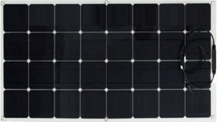Figure 14. PV module

## 15.png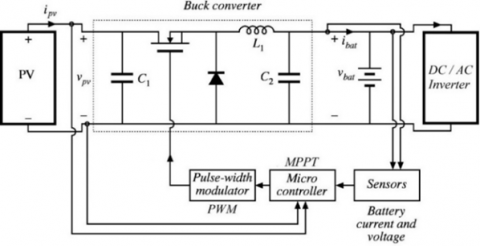Figure 15. The connection circuit of the buck converter with MPPT and battery

For battery recharge, the voltage and current sensors need to be connected to measure the voltage and the current. The battery's terminals are connected to the DC/DC converter's output terminals, and the DC/DC converter's input is connected to the PV module. These last terminals are also connected to monitor voltage, current, and feed the monitored data, whether it is caused by the battery or a PV unit to a controller called MPPT, which helps to produce the gate of the DC/DC converter. Figure 15 illustrates the entire system connection circuit.

A. PV measurements:

Figure 16 shows the measurement data obtained from the solar panel used in the experiment. The experiment was performed under $800 W / m^{2}$ irradiance.

## 16.pngFigure 16. Measured $I-V$ and $P-V$ curves of the VS-100P Module

B. Battery

Table 2 shows the features of the battery used in the experiment to save power.

Table 2. Features of the Ultracell battery

 Type Ultracell – Deep cycle gel Part number UCG100-12 Normal voltage 12 V Normal capacity (10 Hr) 100 AH Internal resistance 5.9 $\mathrm{m} \Omega$ Weight 30.4 kg

C. Buck converter

As illustrated in Figure 17, switched input current $i_{1}(t)$ contains large high-frequency harmonics, hence the inductance of the input loop is critical inductance leading to ringing, voltage spikes, switching loss. The second loop contains a filter inductor, and hence its current $i_{2}(t)$ is nearly DC. Figure 18 illustrates the waveforms of the input current $i_{1}(t)$ and the output current form $i_{2}(t)$.

## 17.pngFigure 17. The circuit of the Buck converter

## 18.png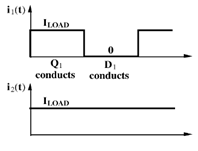Figure 18. Waveforms of currents $i_{1}$ and $i_{2}$

D. MPPT controller

The MPPT circuit which is based on the “ICM- P&O” shown in Figure 19.

## 19.pngFigure 19. MPPT board

E. Results of the experiment

Figure 20 demonstrates the buildup of the output voltage of the PV as it reaches the steady-state operation in 0.32 msec. The curve is over-damped (no overshooting), so it does not require high-power electronics.

## 20.pngFigure 20. The buildup of the PV voltage until it reaches the steady state

## 21.png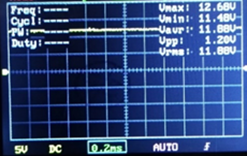Figure 21. The buildup of the PV voltage until it reaches the steady state

## 22.png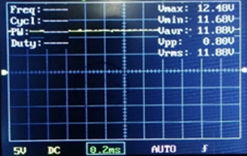Figure 22. The output waveform of the Buck converter (Chargeable voltage)

Figure 21 illustrates the steady-state voltage of the PV. It has a peak-to-peak ripple voltage of 1.2 V, with a maximum of 12.68 V and a minimum of 11.48 V. The average output voltage is measured as 11.88 V.

Figure 22 shows the steady-state voltage of the buck converter. It has a peak-to-peak ripple voltage of 0.8 V, with a maximum of 12.48 V and a minimum of 11.68 V. The average output voltage is calculated as 11.88 V.

6. Conclusions

The adaptive ICM-P&O MPPT algorithm has been proposed to improve the efficiency of PV systems. The algorithm is set up to reduce the major problems that arise in the use of traditional P&O and IC methodologies in dynamic response and steady-state stability.

The algorithm was validated by numerical simulation, considering the experimentally identified PV panels. The implementation of the proposed algorithm showed that the dynamic response is faster compared to the traditional P&O and IC methods, and the stability is also improved. The algorithm is adaptive and also appears to be less sensitive to parameter changes. Moreover, some laboratory tests were performed on a low-power panel to give experimental validity to the effectiveness of the proposed algorithm.

The simulation and experimental results which are fully approved show that the proposed technique can be considered as an effective method for MPPT.

The validity of the introduced ICM-P&O method can be seen in the findings given above. Hence, since the proposed method achieves higher efficiency and faster MPP tracking, the conclusions about the proposed method can be reported.

Besides, due to sudden variations in radiance, the suggested technique leads to limited overshoot, because of which it does not necessitate the use of high-power electronic components.

The experimental system with ICM-P&O MPPT has a high level of performance and can achieve its steady-state at 0.35ms. Because the system works in the damping range, it does not need high-power electronics.

Finally, the contribution of the paper can be summarized on the following:

• The proposed method is a hybrid technique which combine of P&O and ICM method, in which it is characterized by fast response and low output oscillations.
• The proposed method can operate efficiently under severe operating conditions.
• The proposed system is implemented practically and the results match with the theoretical works, which make our system a platform for maximum power point tracker.
References

 Paiano, A. (2015). Photovoltaic waste assessment in Italy. Renewable and Sustainable Energy Reviews, 41: 99-112. https://doi.org/10.1016/j.rser.2014.07.208

 de Wild-Scholten, M.M. (2013). Energy payback time and carbon footprint of commercial photovoltaic systems. Solar Energy Materials and Solar Cells, 119: 296-305. https://doi.org/10.1016/j.solmat.2013.08.037

 Global Market Outlook for Solar Power. https://www.solarpowereurope.org.

 Mahmoudi, S., Huda, N., Alavi, Z., Islam, M.T., Behnia, M. (2019). End-of-life photovoltaic modules: A systematic quantitative literature review. Resources, Conservation and Recycling, 146: 1-16. https://doi.org/10.1016/j.resconrec.2019.03.018

 Chowdhury, M.S., Rahman, K.S., Chowdhury, T., Nuthammachot, N., Techato, K., Akhtaruzzaman, M., Amin, N. (2020). An overview of solar photovoltaic panels’ end-of-life material recycling. Energy Strategy Reviews, 27: 100431. https://doi.org/10.1016/j.esr.2019.100431

 Devabhaktuni, V., Alam, M., Depuru, S.S.S.R., Green II, R.C., Nims, D., Near, C. (2013). Solar energy: Trends and enabling technologies. Renewable and Sustainable Energy Reviews, 19: 555-564. https://doi.org/10.1016/j.rser.2012.11.024

 Esram, T., Chapman, P.L. (2007). Comparison of photovoltaic array maximum power point tracking techniques. IEEE Transactions on Energy Conversion, 22(2): 439-449. https://doi.org/10.1109/TEC.2006.874230

 Reisi, A.R., Moradi, M.H., Jamasb, S. (2013). Classification and comparison of maximum power point tracking techniques for photovoltaic system: A review. Renewable and Sustainable Energy Reviews, 19: 433-443. https://doi.org/10.1016/j.rser.2012.11.052

 Karatepe, E., Hiyama, T. (2009). Artificial neural network-polar coordinated fuzzy controller based maximum power point tracking control under partially shaded conditions. IET Renewable Power Generation, 3(2): 239-253. https://doi.org/10.1049/iet-rpg:20080065

 Miyatake, M., Veerachary, M., Toriumi, F., Fujii, N., Ko, H. (2011). Maximum power point tracking of multiple photovoltaic arrays: A PSO approach. IEEE Transactions on Aerospace and Electronic Systems, 47(1): 367-380. https://doi.org/10.1109/TAES.2011.5705681

 Hadji, S., Krim, F., Gaubert, J.P. (2011). Development of an algorithm of maximum power point tracking for photovoltaic systems using genetic algorithms. In International Workshop on Systems, Signal Processing and Their Applications, WOSSPA, 43-46. https://doi.org/10.1109/WOSSPA.2011.5931408

 Khaehintung, N., Pramotung, K., Tuvirat, B., Sirisuk, P. (2004). RISC-microcontroller built-in fuzzy logic controller of maximum power point tracking for solar-powered light-flasher applications. In 30th Annual Conference of IEEE Industrial Electronics Society, 2004. IECON 2004, 3: 2673-2678. https://doi.org/10.1109/IECON.2004.1432228

 Liao, C.C. (2010). Genetic k-means algorithm based RBF network for photovoltaic MPP prediction. Energy, 35(2): 529-536. https://doi.org/10.1016/j.energy.2009.10.021

 Bauer, J., Wagner, J. M., Lotnyk, A., Blumtritt, H., Lim, B., Schmidt, J., Breitenstein, O. (2009). Hot spots in multicrystalline silicon solar cells: Avalanche breakdown due to etch pits. Physica Status Solidi (RRL)–Rapid Research Letters, 3(2-3): 40-42. https://doi.org/10.1002/pssr.200802250

 Woyte, A., Nijs, J., Belmans, R. (2003). Partial shadowing of photovoltaic arrays with different system configurations: Literature review and field test results. Solar Energy, 74(3): 217-233. https://doi.org/10.1016/S0038-092X(03)00155-5

 Halim, A.A.E.B.A.E., Saad, N.H., El Sattar, A.A. (2019). A comparative study between perturb and observe and cuckoo search algorithm for maximum power point tracking. In 2019 21st International Middle East Power Systems Conference (MEPCON), pp. 716-723. https://doi.org/10.1109/MEPCON47431.2019.9008210

 Hussein, M.S.H. (2015). A modified technique for maximum power point of tracking of photovoltaic system. this thesis was submitted to the graduate school of applied sciences. In Partial Fulfillment of the Requirements for The Degree of Master of Science in Electrical and Electronic Engineering.

 Latran, M.B., Teke, A. (2015). Investigation of multilevel multifunctional grid connected inverter topologies and control strategies used in photovoltaic systems. Renewable and Sustainable Energy Reviews, 42: 361-376. https://doi.org/10.1016/j.rser.2014.10.030

 Manimekalai, P., Harikumar, R., Raghavan, S. (2014). Evaluating the effect of interleaving and maximum power point tracking (MPPT) in boost converter for photo voltaic (PV) power generation system using MATLAB. IJSSST, 14(5): 47-54. https://doi.org/10.5013/IJSSST.a.14.05.07

 Halim, A.A.E.B.A.E., Saad, N.H., El Sattar, A.A. (2019). Application of a combined system between perturb and observe method and incremental conductance technique for MPPT in PV systems. In 2019 21st International Middle East Power Systems Conference (MEPCON), 103-110. https://doi.org/10.1109/MEPCON47431.2019.9008079